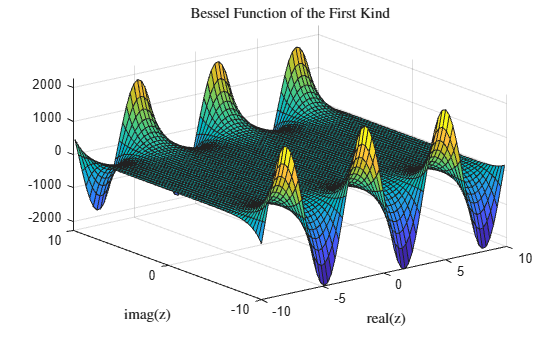# besselj

## 语法

J = besselj(nu,Z)
J = besselj(nu,Z,scale)

## 说明

J = besselj(nu,Z) 为数组 Z 中的每个元素计算第一类 Bessel 函数 Jν(z)。

J = besselj(nu,Z,scale) 指定是否呈指数缩放第一类 Bessel 函数以避免溢出或精度损失。如果 scale 为 1，则 besselj 的输出按因子 exp(-abs(imag(Z))) 进行缩放。

## 示例

z = 0:0.1:20;

J = zeros(5,201); for i = 0:4 J(i+1,:) = besselj(i,z); end

plot(z,J) grid on legend('J_0','J_1','J_2','J_3','J_4','Location','Best') title('Bessel Functions of the First Kind for $\nu \in [0, 4]$','interpreter','latex') xlabel('z','interpreter','latex') ylabel('$J_\nu(z)$','interpreter','latex')$\mathit{z}$ 的复数值计算未缩放的 (J) 和经过缩放的 (Js) 第一类 Bessel 函数 ${\mathit{J}}_{2}\left(\mathit{z}\right)$

x = -10:0.3:10; y = x'; z = x + 1i*y; scale = 1; J = besselj(2,z); Js = besselj(2,z,scale);

surf(x,y,imag(J)) title('Bessel Function of the First Kind','interpreter','latex') xlabel('real(z)','interpreter','latex') ylabel('imag(z)','interpreter','latex')surf(x,y,imag(Js)) title('Scaled Bessel Function of the First Kind','interpreter','latex') xlabel('real(z)','interpreter','latex') ylabel('imag(z)','interpreter','latex')## 输入参数

• 0（默认值）- 无缩放

• 1 - 按 exp(-abs(imag(Z))) 缩放 besselj 的输出

## 详细信息

### Bessel 函数

${z}^{2}\frac{{d}^{2}y}{d{z}^{2}}+z\frac{dy}{dz}+\left({z}^{2}-{\nu }^{2}\right)y=0.$

${J}_{\nu }\left(z\right)={\left(\frac{z}{2}\right)}^{\nu }\sum _{\left(k=0\right)}^{\infty }\frac{{\left(\frac{-{z}^{2}}{4}\right)}^{k}}{k!\Gamma \left(\nu +k+1\right)}\text{\hspace{0.17em}}.$

${Y}_{\nu }\left(z\right)=\frac{{J}_{\nu }\left(z\right)\mathrm{cos}\left(\nu \pi \right)-{J}_{-\nu }\left(z\right)}{\mathrm{sin}\left(\nu \pi \right)}\text{\hspace{0.17em}}.$

## 提示

Bessel 函数与 Hankel 函数相关，也称为第三类 Bessel 函数，

$\begin{array}{l}{H}_{\nu }^{\left(1\right)}\left(z\right)={J}_{\nu }\left(z\right)+i\text{\hspace{0.17em}}{Y}_{\nu }\left(z\right)\\ {H}_{\nu }^{\left(2\right)}\left(z\right)={J}_{\nu }\left(z\right)-i\text{\hspace{0.17em}}{Y}_{\nu }\left(z\right).\end{array}$

${H}_{\nu }^{\left(K\right)}\left(z\right)$besselhJν(z)besseljYν(z)bessely。Hankel 函数同样构成 Bessel 方程的一组基本解（请参阅 besselh）。